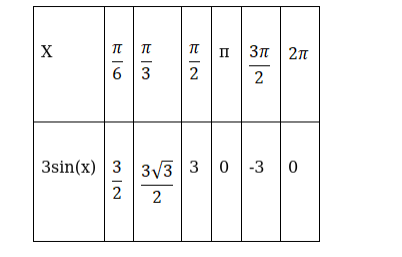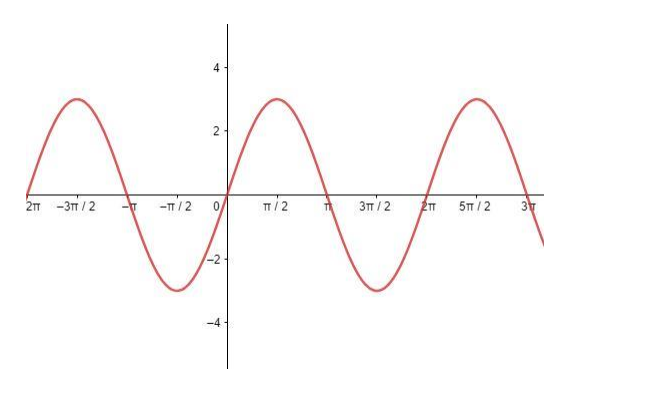# Draw the graph of each of the following functions:`
Question:

Draw the graph of each of the following functions:

3sin x

Solution:

To draw the graph of the curve 3sin(x) assume some standard angle measures which will help in locating the points and drawing the curve.Therefore, the graph of curve 3sin(x) can be drawn asHere, the amplitude of the function sin(x) is increased by 3 times.